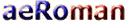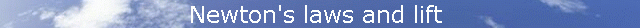So, how does a wing generate lift? To begin to understand lift we must review Newton’s first and third laws. (We will introduce Newton’s second law a little later.) Newton’s first law states: A body at rest will remain at rest, or a body in motion will continue in straight-line motion unless subjected to an external applied force. That means, if one sees a bend in the flow of air, or if air originally at rest is accelerated into motion, a force is acting on it. Newton’s third law states that: For every action there is an equal and opposite reaction. As an example, an object sitting on a table exerts a force on the table (its weight) and the table puts an equal and opposite force on the object to hold it up. In order to generate lift a wing must do something to the air. What the wing does to the air is the action while lift is the reaction. Let’s compare two figures used to show streamlines over a wing. In figure 4 the air comes straight at the wing, bends around it, and then leaves straight behind the wing. We have all seen similar pictures, even in flight manuals. But, the air leaves the wing exactly as it appeared ahead of the wing. There is no net action on the air so there can be no lift! Figure 5 shows the streamlines, as they should be drawn. The air passes over the wing and is bent down. Newton’s first law says that there must be a force on the air to bend it down (the action). Newton’s third law says that there must be an equal and opposite force (up) on the wing (the reaction). To generate lift a wing must divert lots of air down.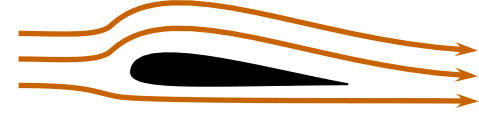Figure 4. Common depiction of airflow over a wing. This wing has no lift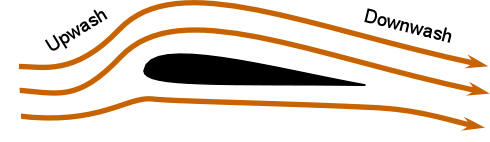Figure 5. True airflow over a wing with lift showing upwash and downwash The lift of a wing is equal to the change in momentum of the air it is diverting down. Momentum is the product of mass and velocity (mv). The most common form of Newton’s second law is F= ma, or force equal mass times acceleration. The law in this form gives the force necessary to accelerate an object of a certain mass. An alternate form of Newton’s second law can be written: The lift of a wing is proportional to the amount of air diverted down times the vertical velocity of that air. It is that simple. For more lift the wing can either divert more air (mass), increase its vertical velocity or a combination of the two. This vertical velocity behind the wing is the vertical component of the "downwash". Figure 6 shows how the downwash appears to the pilot (or in a wind tunnel). The figure also shows how the downwash appears to an observer on the ground watching the wing go by. To the pilot the air is coming off the wing at roughly the angle of attack and at about the speed of the airplane. To the observer on the ground, if he or she could see the air, it would be coming off the wing almost vertically at a relatively slow speed. The greater the angle of attack of the wing the greater the vertical velocity of the air. Likewise, for a given angle of attack, the greater the speed of the wing the greater the vertical velocity of the air. Both the increase in the speed and the increase of the angle of attack increase the length of the vertical velocity arrow. It is this vertical velocity that gives the wing lift.Figure 6. How downwash appears to a pilot and to an observer on the ground As stated, an observer on the ground would see the air going almost straight down behind the plane. This can be demonstrated by observing the tight column of air behind a propeller, a household fan, or under the rotors of a helicopter; all of which are rotating wings. If the air were coming off the blades at an angle the air would produce a cone rather than a tight column. The wing develops lift by transferring momentum to the air. For straight and level flight this momentum eventually strikes the earth. If an airplane were to fly over a very large scale, the scale would weigh the airplane. Let us do a back-of-the-envelope calculation to see how much air a wing might divert. Take for example a Cessna 172 that weighs about 2300 lb (1045 kg). Traveling at a speed of 140 mph (220 km/h), and assuming an effective angle of attack of 5 degrees, we get a vertical velocity for the air of about 11.5 mph (18 km/h) right at the wing. If we assume that the average vertical velocity of the air diverted is half that value we calculate from Newton's second law that the amount of air diverted is on the order of 5 ton/s. Thus, a Cessna 172 at cruise is diverting about five times its own weight in air per second to produce lift. Think how much air is diverted by a 250-ton Boeing 777. Diverting so much air down is a strong argument against lift being just a surface effect (that is only a small amount of air around the wing accounts for the lift), as implied by the popular explanation. In fact, in order to divert 5 ton/sec the wing of the Cessna 172 must accelerate all of the air within 18 feet (7.3 m) above the wing. One should remember that the density of air at sea level is about 2 lb per cubic yard (about 1kg per cubic meter). Figure 7 illustrates the effect of the air being diverted down from a wing. A huge hole is punched through the fog by the downwash from the airplane that has just flown over it.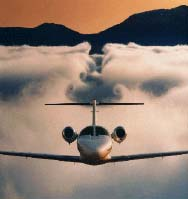Figure 7. Downwash and wing vortices in the fog So how does a thin wing divert so much air? When the air is bent around the top of the wing, it pulls on the air above it accelerating that air downward. Otherwise there would be voids in the air above the wing. Air is pulled from above. This pulling causes the pressure to become lower above the wing. It is the acceleration of the air above the wing in the downward direction that gives lift. (Why the wing bends the air with enough force to generate lift will be discussed in the next section.) Normally, one looks at the air flowing over the wing in the frame of reference of the wing. In other words, to the pilot the air is moving and the wing is standing still. We have already stated that an observer on the ground would see the air coming off the wing almost vertically. But what is the air doing below the wing? Figure 8 shows an instantaneous snapshot of how air molecules are moving as a wing passes by. Remember in this figure the air is initially at rest and it is the wing moving. Arrow "1" will become arrow "2" and so on. Ahead of the leading edge, air is moving up (upwash). At the trailing edge, air is diverted down (downwash). Over the top the air is accelerated towards the trailing edge. Underneath, the air is accelerated forward slightly. Far behind the wing the air is going straight down.Figure 8. Direction of air movement around a wing as seen by an observer on the ground So, why does the air follow this pattern? First, we have to bear in mind that air is considered an incompressible fluid for low-speed flight. That means that it cannot change its volume and that there is a resistance to the formation of voids. Now the air has been accelerated over the top of the wing by of the reduction in pressure. This draws air from in front of the wing and expels if back and down behind the wing. This air must be compensated for, so the air shifts around the wing to fill in. This is similar to the circulation of the water around a canoe paddle. This circulation around the wing is no more the driving force for the lift on the wing than is the circulation in the water drives the paddle. Though, it is true that if one is able to determine the circulation around a wing the lift of the wing can be calculated. Lift and circulation are proportional to each other. One observation that can be made from Figure 8 is that the top surface of the wing does much more to move the air than the bottom. So the top is the more critical surface. Thus, airplanes can carry external stores, such as drop tanks, under the wings but not on top where they would interfere with lift. That is also why wing struts under the wing are common but struts on the top of the wing have been historically rare. A strut, or any obstruction, on the top of the wing would interfere with the lift. MORE (Air Bending Over a Wing) Source: David Anderson, Scott Eberhardt [HOME] [AVIATION] [How Airplanes fly] [History] [Records] [Humor] [News] [Photos] [Stories] [Stamps] [Sounds] [Movies] [HUMANS] [ORIGINS] [SCIENCE] [WEB LINKS] [SITE MAP] [CONTACT]